## Python 实现的随机森林

Random forest is a highly versatile machine learning method with numerous applications ranging from marketing to healthcare and insurance. It can be used to model the impact of marketing on customer acquisition, retention, and churn or to predict disease risk and susceptibility in patients.

Random forest is a capable of regression and classification. It can handle a large number of features, and it's helpful for estimating which or your variables are important in the underlying data being modeled.

This is a post about random forests using Python.

### What is a Random Forest?

Random forest is solid choice for nearly any prediction problem (even non-linear ones). It's a relatively new machine learning strategy (it came out of Bell Labs in the 90s) and it can be used for just about anything. It belongs to a larger class of machine learning algorithms called ensemble methods.

##### Ensemble Learning

Ensemble learning involves the combination of several models to solve a single prediction problem. It works by generating multiple classifiers/models which learn and make predictions independently. Those predictions are then combined into a single (mega) prediction that should be as good or better than the prediction made by any one classifer.

Random forest is a brand of ensemble learning, as it relies on an ensemble of decision trees. More on ensemble learning in Python here: Scikit-Learn docs.

### 什么是随机森林？

##### Randomized Decision Trees

So we know that random forest is an aggregation of other models, but what types of models is it aggregating? As you might have guessed from its name, random forest aggregates Clasification (or Regression) Trees. A decision tree is composed of a series of decisions that can be used to classify an observation in a dataset.

##### Random Forest

The algorithm to induce a random forest will create a bunch of random decision trees automatically. Since the trees are generated at random, most won't be all that meaningful to learning your classification/regression problem (maybe 99.9% of trees).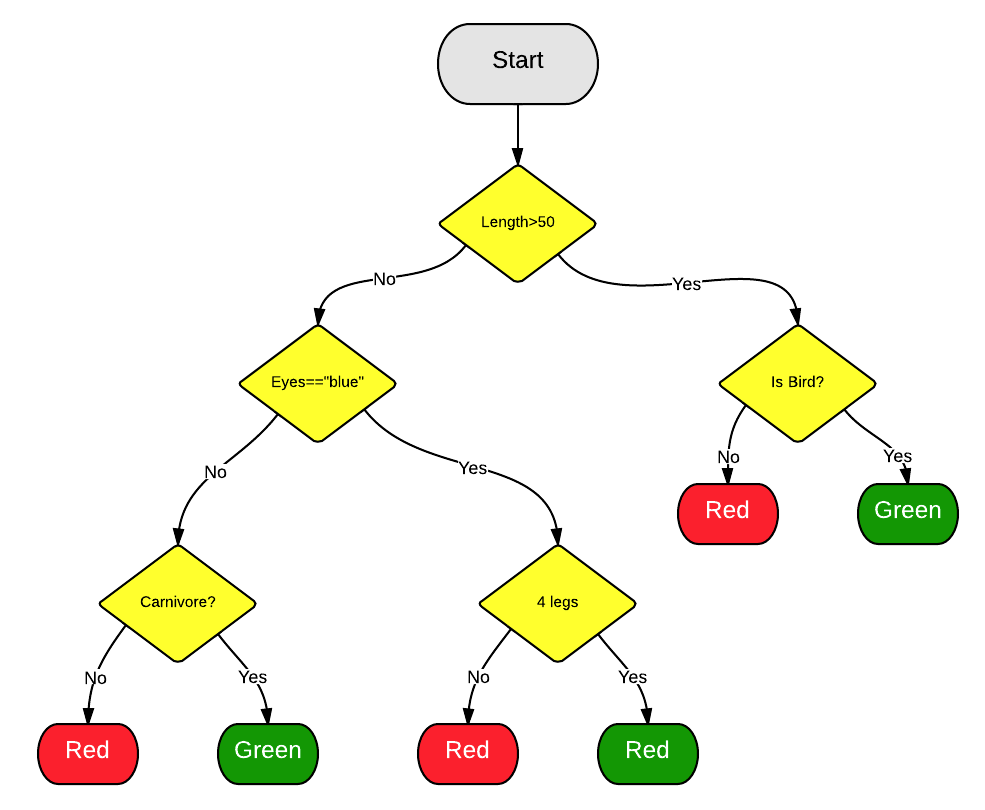If an observation has a length of 45, blue eyes, and 2 legs, it's going to be classified as red.

##### 随机森林##### Arboreal Voting

So what good are 10000 (probably) bad models? Well it turns out that they really aren't that helpful. But what is helpful are the few really good decision trees that you also generated along with the bad ones.

When you make a prediction, the new observation gets pushed down each decision tree and assigned a predicted value/label. Once each of the trees in the forest have reported its predicted value/label, the predictions are tallied up and the mode vote of all trees is returned as the final prediction.

Simply, the 99.9% of trees that are irrelevant make predictions that are all over the map and cancel each another out. The predictions of the minority of trees that are good top that noise and yield a good prediction.##### 树的投票### Why you should I use it?

##### It's Easy

Random forest is the Leatherman of learning methods. You can throw pretty much anything at it and it'll do a serviceble job. It does a particularly good job of estimating inferred transformations, and, as a result, doesn't require much tuning like SVM (i.e. it's good for folks with tight deadlines).

##### An Example Transformation

Random forest is capable of learning without carefully crafted data transformations. Take the thef(x) = log(x)function for example.

Create some fake data and add a little noise.

```import numpy as np
x = np.random.uniform(1, 100, 1000)
y = np.log(x) + np.random.normal(0, .3, 1000)```
full gist here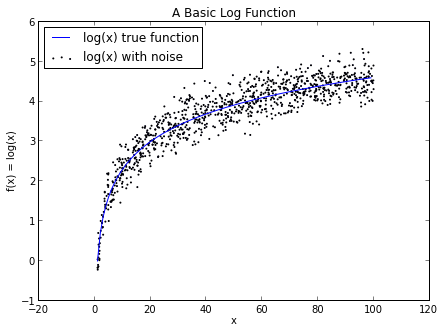### 为什么你让我用它？

##### 简单

[译者注：Leatherman就是那家生产多功能折叠刀的公司，类似瑞士军刀]

##### 一个映射的例子

```import numpy as np
x = np.random.uniform(1, 100, 1000)
y = np.log(x) + np.random.normal(0, .3, 1000)```
full gist hereIf we try and build a basic linear model to predictyusingxwe wind up with a straight line that sort of bisects thelog(x)function. Whereas if we use a random forest, it does a much better job of approximating thelog(x)curve and we get something that looks much more like the true function.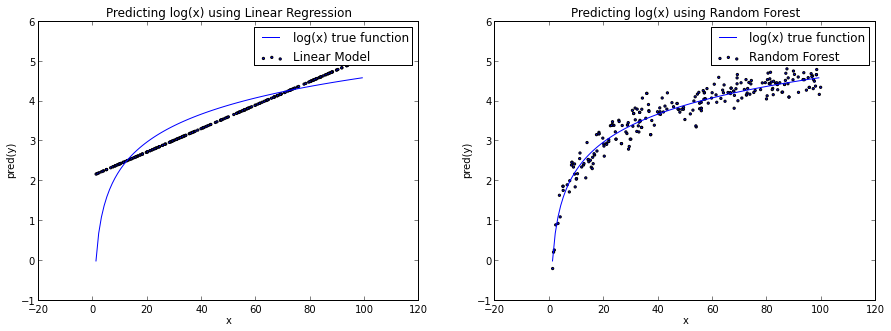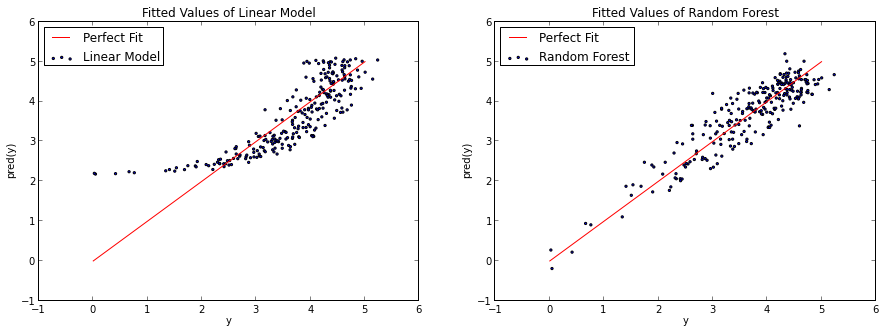You could argue that the random forest overfits thelog(x)function a little bit. Either way, I think this does a nice job of illustrating how the random forest isn't bound by linear constraints.### Uses

##### Variable Selection

One of the best use cases for random forest is feature selection. One of the byproducs of trying lots of decision tree variations is that you can examine which variables are working best/worst in each tree.

When a certain tree uses a one variable and another doesn't, you can compare the value lost or gained from the inclusion/exclusion of that variable. The good random forest implementations are going to do that for you, so all you need to do is know which method or variable to look at.

In the following examples, we're trying to figure out which variables are most important for classifying a wine as being red or white.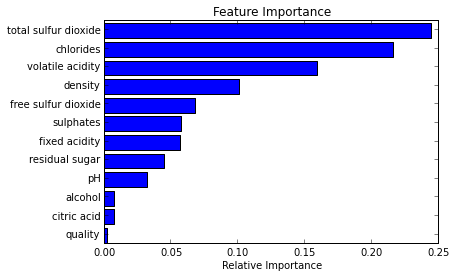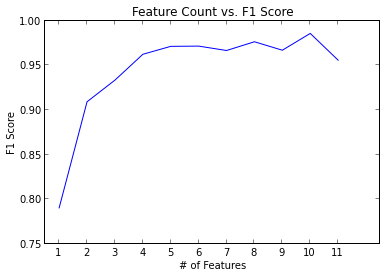### 使用

##### 变量选择Classification

Random forest is also great for classification. It can be used to make predictions for categories with multiple possible values and it can be calibrated to output probabilities as well. One thing you do need to watch out for is overfitting. Random forest can be prone to overfitting, especially when working with relatively small datasets. You should be suspicious if your model is making "too good" of predictions on our test set.

One way to overfitting is to only use really relevant features in your model. While this isn't always cut and dry, using a feature selection technique (like the one mentioned previously) can make it a lot easier.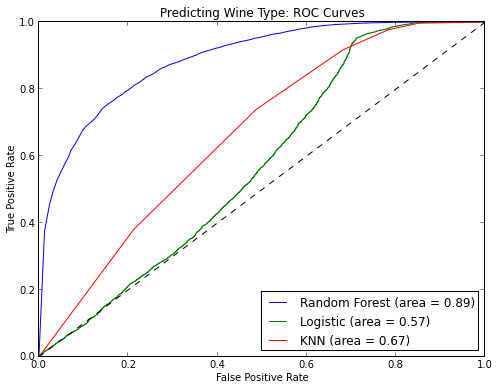##### Regression

Yep. It does regression too.

I've found that random forest--unlike other algorithms--does really well learning on categorical variables or a mixture of categorical and real variables. Categorical variables with high cardinality (# of possible values) can be tricky, so having something like this in your back pocket can come in quite useful.### A Short Python Example

Scikit-Learn is a great way to get started with random forest. The scikit-learn API is extremely consistent across algorithms, so you horse race and switch between models very easily. A lot of times I start with something simple and then move to random forest.

One of the best features of the random forest implementation in scikit-learn is then_jobsparameter. This will automatically paralellize fitting your random forest based on the number of cores you want to use. Here's a great presentation by scikit-learn contributor Olivier Grisel where he talks about training a random forest on a 20 node EC2 cluster.

```from sklearn.datasets import load_iris
from sklearn.ensemble import RandomForestClassifier
import pandas as pd
import numpy as np

iris = load_iris()
df = pd.DataFrame(iris.data, columns=iris.feature_names)
df['is_train'] = np.random.uniform(0, 1, len(df)) <= .75
df['species'] = pd.Factor(iris.target, iris.target_names)
df.head()

train, test = df[df['is_train']==True], df[df['is_train']==False]

features = df.columns[:4]
clf = RandomForestClassifier(n_jobs=2)
y, _ = pd.factorize(train['species'])
clf.fit(train[features], y)

preds = iris.target_names[clf.predict(test[features])]
pd.crosstab(test['species'], preds, rownames=['actual'], colnames=['preds'])```

Looks pretty good!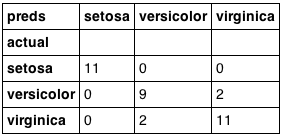### Final Thoughts

Random forests are remarkabley easy to use given how advanced they are. As with any modeling, be wary of overfitting. If you're interested in getting started with random forest inR, check out the randomForest package.

### 一个简短的python例子

Scikit-Learn是开始使用随机森林的一个很好的方式。scikit-learn API在所以算法中极其的一致，所有你测试和在不同的模型间切换非常容易。很多时候，我从一些简单的东西开始，然后转移到了随机森林。

```from sklearn.datasets import load_iris
from sklearn.ensemble import RandomForestClassifier
import pandas as pd
import numpy as np

iris = load_iris()
df = pd.DataFrame(iris.data, columns=iris.feature_names)
df['is_train'] = np.random.uniform(0, 1, len(df)) <= .75
df['species'] = pd.Factor(iris.target, iris.target_names)
df.head()

train, test = df[df['is_train']==True], df[df['is_train']==False]

features = df.columns[:4]
clf = RandomForestClassifier(n_jobs=2)
y, _ = pd.factorize(train['species'])
clf.fit(train[features], y)

preds = iris.target_names[clf.predict(test[features])]
pd.crosstab(test['species'], preds, rownames=['actual'], colnames=['preds'])```### 结语